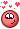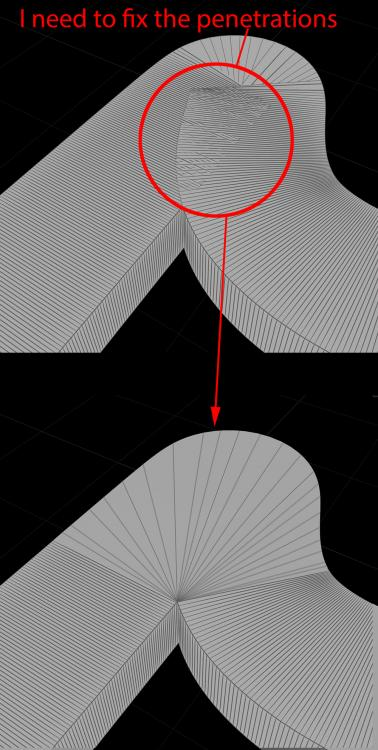## Recommended Posts

Hi guys;

I tried to deform a box using "GameDev Path Deform" as you can see in the image below which works fine but how can I fix the artifacts (penetrations) at the overlapping areas?

(I mean I need to fix the penetrations and make a clean topology).

Thanks for helping.

Edited by Masoud

##### Share on other sites

Hip HI @Masoud   Ask nowHOPE IT HELPS !

Edited by Librarian
•1

##### Share on other sites

Hello Tesan;

Want to thank you for your quick reply, but I need to fix the penetrations (I mean not curve's "banking").

Here is what I need to fix:##### Share on other sites

You mean Like Weld Points to specific Angle ..Maybe if you ramp you UPSS @Masoud

I have This in our Selectione OF ODforce unde N and Up
HAve FUn ..Asia FORUM

CONTROLL.hiplc

##### Share on other sites

100 % Its possible .I'm just not qualified for that Task..I can visualize and draw in mine Head  ..but lack of the knowledge  ..

someone maybe gonna HelpMaybe if we use this code for intersection inside Path deform and make something different

```//verti
vector getIntersection(vector A;vector B;vector C;vector D;int test)
{
if(A==B || C==D) return B;
vector n1 = normalize(B-A);
vector n2 = normalize(D-C);

if(abs(1.0-abs(dot(n1,n2)))<1e-5){
test = 0;
return B;
}

vector AC = C - A;
float d1 = ( dot(n1,AC) - dot(n2,AC)*dot(n1,n2) ) / ( 1 - dot(n1,n2)*dot(n1,n2) );
float d2 = ( -dot(n2,AC) + dot(n1,AC)*dot(n1,n2) ) / ( 1 - dot(n1,n2)*dot(n1,n2) );

if(d1<0 || d2<0){test=0;return B;}
else if(d2>distance(C,D)){test=0;return B;}

vector p1,p2;
p1 = A + d1*n1;
p2 = C + d2*n2;
test = 1;
return p2;
}

int listPoints1[] = primpoints(1, 0);
vector A = point(1,"P",listPoints1);
vector B = point(1,"P",listPoints1);
int curPrimOnC= vertexprim(0,@vtxnum);
int curPrimOnD= vertexprim(0,@vtxnum+1);
if(curPrimOnC!=curPrimOnD){
removepoint(0,vertexpoint(0,@vtxnum));
return;
}
vector C = point(0,"P",vertexpoint(0,@vtxnum));
vector D = point(0,"P",vertexpoint(0,@vtxnum+1));
vector p=C;
int test = 0;
p = getIntersection(A,B,C,D,test);
removepoint(0,vertexpoint(0,@vtxnum));
if(test==1){
}```
```//prim
vector getIntersection(vector A;vector B;vector C;vector D)
{
if(A==B || C==D) return B;
vector n1 = normalize(B-A);
vector n2 = normalize(D-C);

if(abs(1.0-abs(dot(n1,n2)))<1e-5) return B;

vector AC = C - A;
float d1 = ( dot(n1,AC) - dot(n2,AC)*dot(n1,n2) ) / ( 1 - dot(n1,n2)*dot(n1,n2) );
float d2 = ( -dot(n2,AC) + dot(n1,AC)*dot(n1,n2) ) / ( 1 - dot(n1,n2)*dot(n1,n2) );

if(d1<0 || d2<0) return B;
else if(d2>distance(C,D)) return B;

vector p1,p2;
p1 = A + d1*n1;
p2 = C + d2*n2;
return p2;
}

int listPoints1[] = primpoints(0, @primnum);
vector A = point(0,"P",listPoints1);
vector B = point(0,"P",listPoints1);
vector p=B;
float minimumDist = 1e+6;
for(int i=0;i<nprimitives(1);i++){
int listLinearVertices[] = primvertices(1, i);
for(int curIndex=0;curIndex<len(listLinearVertices);curIndex++){
int curPrimOnC = vertexprim(1,listLinearVertices[curIndex]);
int nextIndex = curIndex+1;
int curPrimOnD = vertexprim(1,listLinearVertices[nextIndex]);
if(curPrimOnC!=curPrimOnD){
continue;
}
vector C = point(1,"P",vertexpoint(1,listLinearVertices[curIndex]));
vector D = point(1,"P",vertexpoint(1,listLinearVertices[nextIndex]));
vector outP = getIntersection(A,B,C,D);
if(outP!=B && distance(B,outP)<minimumDist){
p = outP;
minimumDist = distance(B,outP);
}
}
}
removeprim(0, @primnum, 1);

It works if  You make poly expand before Path deformEdited by Librarian

## Create an account

Register a new account